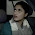## HOW TO FIND AREA OF THE CIRCLE WHICH IS INTERIOR TO THE PARABOLA

HOW TO FIND AREA OF THE CIRCLE  WHICH IS INTERIOR TO THE PARABOLA

## Area Under Curves

Let  us write two equations of circle and parabola respectively
4x2+ 4y2  = 9 ------------------- (1)

and x2      = 4y    -------------------(2)

Reducing (1) to standard form by dividing 4 .we get
x2y2  (3/2)2
Ist of all draw figures of both the circle and the parabola in cartesian plane.

As it can be seen from figure both  curves intersect each other at two points say A and A' .
Next we have to find these two coordinates points of intersection . Solving (1) and (2) to find the values of x and y
Putting  the value of ' x2 from (2) in (1) we get

4(4y)+ 4y2 = 9

16y + 4y2 - 9 = 0

4y2 - 16y -9 = 0
y = (-16+20)/8  and (-16-20)/8
y = 1/2  and -9/2

So Rejecting the -ve value of y ,because when we put negative value (-9/2) in eq (2) , we shall have two complex values of "x" which are not acceptable.
so only put positive value (1/2) of   'y'  in (2) we get two real values of  'x' such that    x= 土⇃2,
Now we can write coordinate M(⇃2,0) and N (-⇃2,0)

= 2 × Area OBAO
Note this step carefully

Multiplying every terms with 2 which is written at  beginning  of the previous line.

Putting the values of upper and lower limits of x

## Final words

Thanks for visiting this website and spending your valuable time to read this post.If you liked this post , do share it with your friends to benefit them also we shall meet in next post , till then bye and take care......

Share:

1.Nice post. I learned some new information. Thanks for sharing.

Article submission sites
Guest posting sites

2.great post. I learned some new information. Thanks for sharing.Tech Guest Post Free

3.great post love it SEO write for us

4.you have a question about a new product,Write For Us Application Development